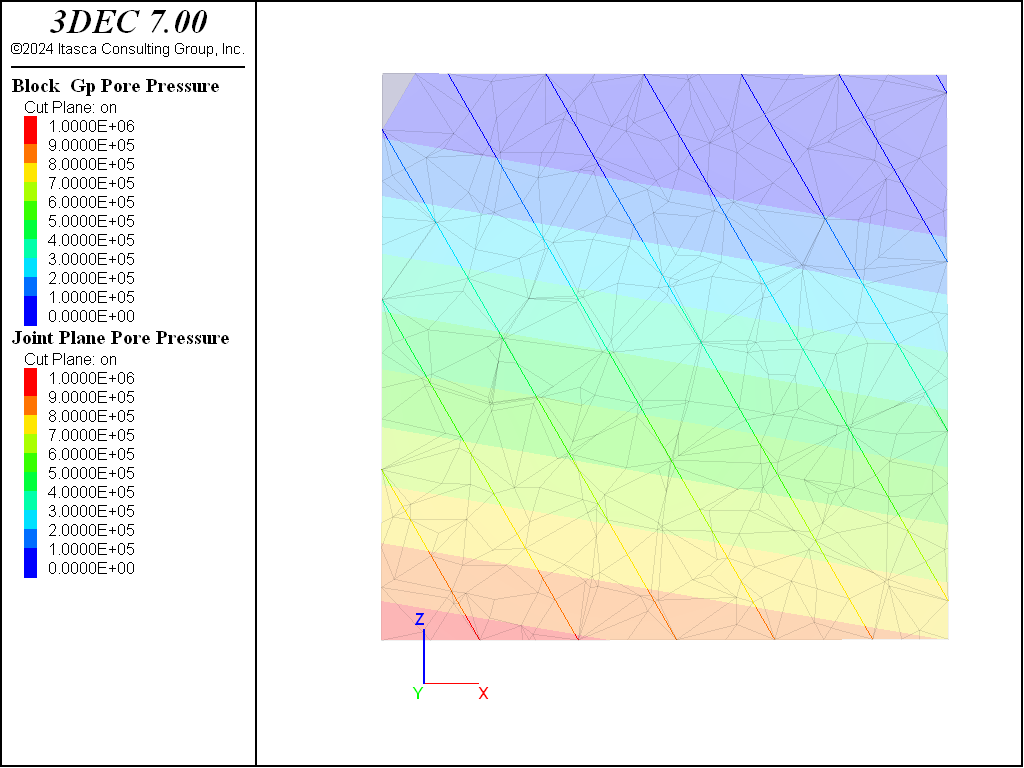# Specifying Initial Pore Pressure

Pore pressures may be initialized in the same way as stresses, namely by using the block insitu command. To assign pore pressures, simply use the pore-pressure keyword. Usually a gradient is also supplied with the gradient keyword. As with stress initialization, the specified value will be the pore pressure at the origin (0,0,0) and the gradient is the change in pore pressure per unit length in the $$x$$, $$y$$, and $$z$$ directions. The code below shows an example:

block insitu pore-pressure 1e6 gradient 0 0 9810


Note that 3DEC assumes that the material is always saturated — there is no partially saturated flow. Pore pressures above the water table are 0. Also note that pore pressures are actually assigned to gridpoints, and that pore pressures in zones are the average of four gridpoint pore pressures. Pore pressures in the zones are used to calculate effective stresses, which are used to determine zone failure.

Pore pressures are assigned to joint contacts with the same command. The effective normal stress on the joint is used to determine contact failure.

It is also possible to specify pore pressures with the block water command. If the fluid density and the gravity are given, the pore pressures will be automatically calculated based on the location of a water table. The water table can be defined either as a plane, or as a geometric surface (e.g., a dxf). A simple example is shown below.

model new
model random 10000

block create brick 0,100  0,100  0,100

block cut joint-set dip 60 dip-direction 90 spacing 15

block zone generate edgelength 10

model gravity 0 0 -9.81

block water density 1000

; 10 degree dipping water table
block water plane normal 0.17,0,0.98 origin 50,50,90Figure 1: Pore pressure in zones (semi-transparent) and joints calculated by the block water command.

More details about assigning pore pressures and their effect on the model can be found in i Pore Pressure and Effective Stress.

This discussion assumes the model is not run with model configure fluid on, and there are not flow calculations. For more information about specifying initial pore pressures when doing flow calculations, see the i Fluid manual in the Theory and Background section.